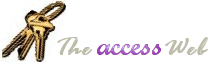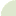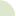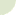Carte du site  Remerciements  Netiquette  Bugs  Tables  Requêtes  Formulaires  États (rapports)  Modules  APIs  Chaînes  Date/Time  Général  Ressources  Téléchargeables  Termes d'usageModules: Convertir un montant (\$500) en mots (Five Hundred Dollars) Author(s)Joe FosterNDLT: La conversion en mots est en anglais.

(Q)    I'm looking to have Access convert the currency value "\$531.20" into the text "Five hundred thirty one and twenty cents."

(A)    Use the following function provided by Joe Foster to convert the Currency into English words.

```'************ Code Start **********
'This code was originally written by Joe Foster.
'It is not to be altered or distributed,
'except as part of an application.
'You are free to use it in any application,
'provided the copyright notice is left unchanged.
'
'Code Courtesy of
'Joe Foster
'
' Convert a currency value into an (American) English string
Function English (ByVal N As Currency) As String
Const Thousand = 1000@
Const Million = Thousand * Thousand
Const Billion = Thousand * Million
Const Trillion = Thousand * Billion

If (N = 0@) Then English = "zero": Exit Function

Dim Buf As String: If (N < 0@) Then Buf = "negative " Else Buf = ""
Dim Frac As Currency: Frac = Abs(N - Fix(N))
If (N < 0@ Or Frac <> 0@) Then N = Abs(Fix(N))
Dim AtLeastOne As Integer: AtLeastOne = N >= 1

If (N >= Trillion) Then
Debug.Print N
Buf = Buf & EnglishDigitGroup(Int(N / Trillion)) & " trillion"
N = N - Int(N / Trillion) * Trillion ' Mod overflows
If (N >= 1@) Then Buf = Buf & " "
End If

If (N >= Billion) Then
Debug.Print N
Buf = Buf & EnglishDigitGroup(Int(N / Billion)) & " billion"
N = N - Int(N / Billion) * Billion ' Mod still overflows
If (N >= 1@) Then Buf = Buf & " "
End If

If (N >= Million) Then
Debug.Print N
Buf = Buf & EnglishDigitGroup(N \ Million) & " million"
N = N Mod Million
If (N >= 1@) Then Buf = Buf & " "
End If

If (N >= Thousand) Then
Debug.Print N
Buf = Buf & EnglishDigitGroup(N \ Thousand) & " thousand"
N = N Mod Thousand
If (N >= 1@) Then Buf = Buf & " "
End If

If (N >= 1@) Then
Debug.Print N
Buf = Buf & EnglishDigitGroup(N)
End If

If (Frac = 0@) Then
Buf = Buf & " exactly"
ElseIf (Int(Frac * 100@) = Frac * 100@) Then
If AtLeastOne Then Buf = Buf & " and "
Buf = Buf & Format\$(Frac * 100@, "00") & "/100"
Else
If AtLeastOne Then Buf = Buf & " and "
Buf = Buf & Format\$(Frac * 10000@, "0000") & "/10000"
End If

English = Buf
End Function

' Support function to be used only by English()
Private Function EnglishDigitGroup (ByVal N As Integer) As String
Const Hundred = " hundred"
Const One = "one"
Const Two = "two"
Const Three = "three"
Const Four = "four"
Const Five = "five"
Const Six = "six"
Const Seven = "seven"
Const Eight = "eight"
Const Nine = "nine"
Dim Buf As String: Buf = ""
Dim Flag As Integer: Flag = False

'Do hundreds
Select Case (N \ 100)
Case 0: Buf = "":  Flag = False
Case 1: Buf = One & Hundred: Flag = True
Case 2: Buf = Two & Hundred: Flag = True
Case 3: Buf = Three & Hundred: Flag = True
Case 4: Buf = Four & Hundred: Flag = True
Case 5: Buf = Five & Hundred: Flag = True
Case 6: Buf = Six & Hundred: Flag = True
Case 7: Buf = Seven & Hundred: Flag = True
Case 8: Buf = Eight & Hundred: Flag = True
Case 9: Buf = Nine & Hundred: Flag = True
End Select

If (Flag <> False) Then N = N Mod 100
If (N > 0) Then
If (Flag <> False) Then Buf = Buf & " "
Else
EnglishDigitGroup = Buf
Exit Function
End If

'Do tens (except teens)
Select Case (N \ 10)
Case 0, 1: Flag = False
Case 2: Buf = Buf & "twenty": Flag = True
Case 3: Buf = Buf & "thirty": Flag = True
Case 4: Buf = Buf & "forty": Flag = True
Case 5: Buf = Buf & "fifty": Flag = True
Case 6: Buf = Buf & "sixty": Flag = True
Case 7: Buf = Buf & "seventy": Flag = True
Case 8: Buf = Buf & "eighty": Flag = True
Case 9: Buf = Buf & "ninety": Flag = True
End Select

If (Flag <> False) Then N = N Mod 10
If (N > 0) Then
If (Flag <> False) Then Buf = Buf & "-"
Else
EnglishDigitGroup = Buf
Exit Function
End If

'Do ones and teens
Select Case (N)
Case 0: ' do nothing
Case 1: Buf = Buf & One
Case 2: Buf = Buf & Two
Case 3: Buf = Buf & Three
Case 4: Buf = Buf & Four
Case 5: Buf = Buf & Five
Case 6: Buf = Buf & Six
Case 7: Buf = Buf & Seven
Case 8: Buf = Buf & Eight
Case 9: Buf = Buf & Nine
Case 10: Buf = Buf & "ten"
Case 11: Buf = Buf & "eleven"
Case 12: Buf = Buf & "twelve"
Case 13: Buf = Buf & "thirteen"
Case 14: Buf = Buf & "fourteen"
Case 15: Buf = Buf & "fifteen"
Case 16: Buf = Buf & "sixteen"
Case 17: Buf = Buf & "seventeen"
Case 18: Buf = Buf & "eighteen"
Case 19: Buf = Buf & "nineteen"
End Select

EnglishDigitGroup = Buf
End Function
'************ Code End **********

```© 1998-2001, Dev Ashish, All rights reserved. Optimized for Microsoft Internet Explorer